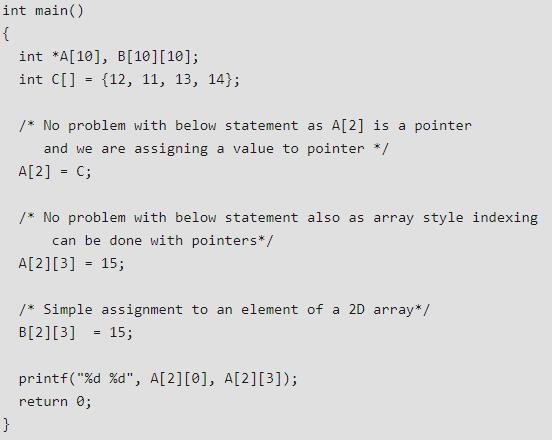Courses

# Test: Arrays - 1

## 10 Questions MCQ Test Learn to Program with C++: Beginner to Expert | Test: Arrays - 1

Description
This mock test of Test: Arrays - 1 for Back-End Programming helps you for every Back-End Programming entrance exam. This contains 10 Multiple Choice Questions for Back-End Programming Test: Arrays - 1 (mcq) to study with solutions a complete question bank. The solved questions answers in this Test: Arrays - 1 quiz give you a good mix of easy questions and tough questions. Back-End Programming students definitely take this Test: Arrays - 1 exercise for a better result in the exam. You can find other Test: Arrays - 1 extra questions, long questions & short questions for Back-End Programming on EduRev as well by searching above.
QUESTION: 1

### Which of the following correctly declares an array?

Solution:

Because array variable and values need to be declared after the datatype only.

QUESTION: 2

Solution:
QUESTION: 3

### What is output? # include <stdio.h>   void print(int arr[]) {    int n = sizeof(arr) / sizeof(arr);    int i;    for (i = 0; i < n; i++)       printf("%d ", arr[i]); }   int main() {    int arr[] = {1, 2, 3, 4, 5, 6, 7, 8};    print(arr);    return 0; }

Solution:
QUESTION: 4

Which of the following accesses the seventh element stored in array?

Solution:

The array location starts from zero, So it can accessed by array.

QUESTION: 5

What will be the output of the following code?

#include"stdio.h"
void main()
{
int a;
printf("%d %d", a[-1], a);
}

Solution:
QUESTION: 6

Assume the following C variable declaration
int *A , B;
Of the following expressions I A II A III B IV B which will not give compile-time errors if used as left hand sides of assignment statements in a C program (GATE CS 2003)?

Solution:QUESTION: 7

What is the output of this program?

#include < stdio.h >

using namespace std;

int main()

{

int array[] = {10, 20, 30};

cout << -2[array];

return 0;

}

Solution:

It’s just printing the negative value of the concern element.

QUESTION: 8

What is the maximum number of dimensions an array in C may have?

Solution:
QUESTION: 9

Predict the output of the below program:

#include <stdio.h>
#define SIZE(arr) sizeof(arr) / sizeof(*arr);

void fun(int* arr, int n)

{
int i;
*arr += *(arr + n - 1) += 10;
}

void printArr(int* arr, int n)

{
int i;
for(i = 0; i < n; ++i)
printf("%d ", arr[i]);
}

int main()

{

int arr[] = {10, 20, 30};
int size = SIZE(arr);
fun(arr, size);
printArr(arr, size);
return 0;

}

Solution:

The crux of the question lies in the expression: *arr += *(arr + n - 1) += 10; The composite operator (here +=) has right to left associativity. First 10 is added to the last element of the array. The result is then added to the first element of the array.

QUESTION: 10

What is the index number of the last element of an array with 9 elements?

Solution:
• So here the last element is 9 so the index will be

size =9;

array-index of last element is = 9-1

=  8.